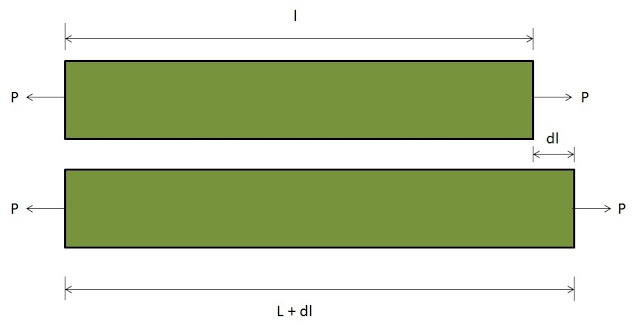# what is strain in FEA?

Questions Category: Mechanical engineering what is strain in FEA?

strain= (change in length)/(original length)
e= dl/l

When an external force is applied on a body, there is some change occur in the dimension of the body. The ratio of this change of dimension in the body to its actual length is called strain.

For example: if you have a bar of length l and an external force P is applied on the bar, then there is some change in the length of the bar. Let the change produced in the bar is dl. Then the strain is the ratio of this change in the length to the original (actual) length. Strain is dimensionless quantity.When an external force is applied on a body, there is some change occur in the dimension of the body. The ratio of this change of dimension in the body to its actual length is called strain.

For example: if you have a bar of length l and an external force P is applied on the bar, then there is some change in the length of the bar. Let the change produced in the bar is dl. Then the strain is the ratio of this change in the length to the original (actual) length. Strain is dimensionless quantity.

Mathematically,

strain= (change in length)/(original length)
e= dl/l

Find NULLED WordPress Themes and Plugins at NulledHub.net